# JP5310603B2 - Mobile device and power control method - Google Patents

## Info

Publication number
JP5310603B2
JP5310603B2 JP2010048687A JP2010048687A JP5310603B2 JP 5310603 B2 JP5310603 B2 JP 5310603B2 JP 2010048687 A JP2010048687 A JP 2010048687A JP 2010048687 A JP2010048687 A JP 2010048687A JP 5310603 B2 JP5310603 B2 JP 5310603B2
Authority
JP
Japan
Prior art keywords
unit
threshold
signal
value
mobile device
Prior art date
Legal status (The legal status is an assumption and is not a legal conclusion. Google has not performed a legal analysis and makes no representation as to the accuracy of the status listed.)
Active
Application number
JP2010048687A
Other languages
Japanese (ja)
Other versions
JP2011188053A (en
Inventor

Original Assignee

Priority date (The priority date is an assumption and is not a legal conclusion. Google has not performed a legal analysis and makes no representation as to the accuracy of the date listed.)
Filing date
Publication date
Application filed by 富士通株式会社 filed Critical 富士通株式会社
Priority to JP2010048687A priority Critical patent/JP5310603B2/en
Publication of JP2011188053A publication Critical patent/JP2011188053A/en
Application granted granted Critical
Publication of JP5310603B2 publication Critical patent/JP5310603B2/en
Application status is Active legal-status Critical
Anticipated expiration legal-status Critical

• 230000001276 controlling effects Effects 0 abstract 1

## Images

•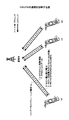•••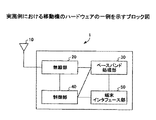•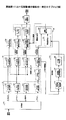•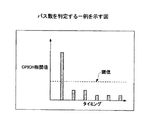••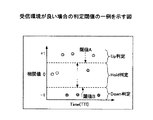•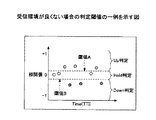•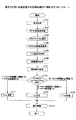•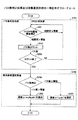•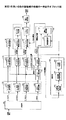•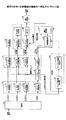•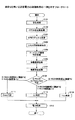•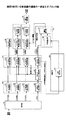•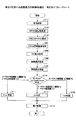•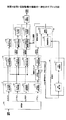•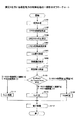•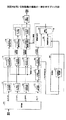•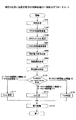•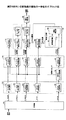•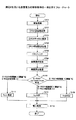•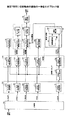•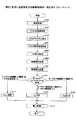•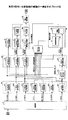•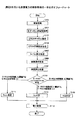•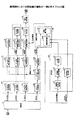•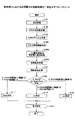## Classifications

• HELECTRICITY
• H04ELECTRIC COMMUNICATION TECHNIQUE
• H04WWIRELESS COMMUNICATION NETWORKS
• H04W52/00Power management, e.g. TPC [Transmission Power Control], power saving or power classes
• H04W52/04TPC
• H04W52/06TPC algorithms
• H04W52/08Closed loop power control
• HELECTRICITY
• H04ELECTRIC COMMUNICATION TECHNIQUE
• H04WWIRELESS COMMUNICATION NETWORKS
• H04W52/00Power management, e.g. TPC [Transmission Power Control], power saving or power classes
• H04W52/04TPC
• H04W52/06TPC algorithms
• H04W52/12Outer and inner loops
• HELECTRICITY
• H04ELECTRIC COMMUNICATION TECHNIQUE
• H04WWIRELESS COMMUNICATION NETWORKS
• H04W52/00Power management, e.g. TPC [Transmission Power Control], power saving or power classes
• H04W52/04TPC
• H04W52/06TPC algorithms
• HELECTRICITY
• H04ELECTRIC COMMUNICATION TECHNIQUE
• H04WWIRELESS COMMUNICATION NETWORKS
• H04W52/00Power management, e.g. TPC [Transmission Power Control], power saving or power classes
• H04W52/04TPC
• H04W52/18TPC being performed according to specific parameters
• H04W52/24TPC being performed according to specific parameters using SIR [Signal to Interference Ratio] or other wireless path parameters

## Abstract

A mobile terminal includes a receiving part configured to receive from a base station a reception signal including a control signal used for controlling transmission power; a comparing part configured to compare a correlation value, which is obtained from the control signal included in the reception signal and a first unique signal, with a threshold; a control part configured to control the transmission power based on a comparison result of the comparing part; and a threshold generating part configured to change the threshold according to a reception environment.

## Description

The present invention relates to a mobile device that performs wireless communication, for example, a mobile device that performs wireless communication by wireless such as W-CDMA (Wideband-Code Division Multiple Access).

The W-CDMA system is one of wireless communication interfaces defined by IMT-2000 (International Mobile Telecommunications-2000), and is positioned as the most mainstream wireless communication system. W-CDMA enables multimedia access of voice, moving images, data, etc. at a maximum transmission rate of 384 Kbps.

In recent years, research and development of wireless communication methods called HSDPA (High Speed Downlink Packet Access) and HSUPA (High Speed Uplink Packet Access) based on the W-CDMA technology have been advanced.

FIG. 1 is a diagram for explaining HSUPA communication. Communication is performed by HSUPA during uplink transmission from a UE (User Equipment) to a base station. The UE transmits SI (Scheduling Information) as an uplink data transmission request to the base station. Information related to transmission data to be transmitted by the UE is mapped to the SI. For example, “highest priority logical channel ID”, “total logical channel data amount”, “highest priority logical channel data amount”, “transmission that can be transmitted by the UE” "Power" information is mapped.

Uplink E-DPCCH (E-DCH Dedicated Physical Control Channel) is mapped with information on uplink data, and E-TFCI (E-DCH Transport Format Combination Indicator), RSN (Retransmission Sequence Number), and Happybit are mapped. The

The base station aggregates a plurality of SIs sent from the UE, and performs scheduling for determining the transmission timing of the UE that performs uplink transmission based on the communication quality of the UE, the priority of the uplink data, and the like. After scheduling, the base station transmits Grant to the UE as permission for uplink transmission. There are two types of grants: absolute grant and relative grant, and the absolute grant is transmitted by downlink E-AGCH (E-DCH Absolute Grant Channel). The base station notifies each UE of the maximum power that each UE is allowed to transmit. In addition, the relative grant is transmitted by downlink E-RGCH (E-DCH Relative Grant Channel). The base station determines whether the power transmitted by each UE is increased, decreased, or maintained from the present, and uses “Up (increase), Down (decrease), or Hold” as a signal component to each UE. Notice.

After the UE is permitted to perform uplink transmission based on Grant, the UE transmits high-speed uplink access by transmitting user information to the base station through a dedicated channel called E-DCH (Enhanced Dedicated Channel). In the HSUPA system, retransmission control processing is performed in the same manner as the HSDPA system, and the UE receives E-DCH ACK or NACK information by downlink E-HICH (E-DCH HARQ Acknowledgment Indicator Channel).

HSUPA uses the E-RGCH signal component “Up, Down, or Hold” to transmit a UE having an uplink transmission request so that the received power of the E-DCH of the base station becomes a desired throughput. Instruct to increase, maintain or decrease power. The UE determines the transmission power of the uplink E-DCH according to the “Up, Down, or Hold” signal of the E-RGCH. The E-RGCH is transmitted from the base station by multiplying the signature pattern unique to the E-RGCH. The UE determines which command in Table 1 is used to transmit the E-RGCH by calculating a correlation value between the signature pattern unique to the E-RGCH and the received E-RGCH signal.

Here, on the UE side, the E-RGCH correlation value is compared with a threshold value in order to determine “Up, Down, or Hold”. For example, the following E-RGCH signal determination conditions are used.
UP: CE-RGCH > Th
DOWN: CE-RGCH <-Th
HOLD: -Th ≦ CE-RGCH ≦ Th
C E-RGCH is a correlation value between the received E-RGCH and the signature pattern of E-RGCH, and Th is a threshold of E-RGCH. The threshold value Th is set to an appropriate value based on, for example, experiments before shipping the mobile device.

3GPP TS 25.211, 5.3.2.4 E-DCH Relative Grant Channel 3GPP TS 25.212, Mapping for E RGCH Relative Grant

The threshold Th is, for example, a value set in advance so that Up, Down, or Hold can be identified even in a poor reception environment. In an environment where the reception environment is bad, the correlation value between the received E-RGCH and the unique signature pattern of the E-RGCH is small, and the threshold value is also small to determine Up, Down, or Hold with this correlation value. Must be set.

FIG. 2 is a diagram illustrating an example of a correlation value and a threshold when the reception environment is bad. As shown in FIG. 2, when the reception environment is bad, the correlation value with the signature pattern of E-RGCH is small, and thus the threshold values A and B are also set to small absolute values. Hereinafter, although the absolute values of the thresholds A and B are the same, they are not necessarily the same.

On the other hand, since the E-RGCH Hold signal is not transmitted, the correlation with the signature pattern is low. However, since the non-transmission state is a random pattern, the correlation between the random pattern and the signature pattern may be slightly stronger. In this case, even in the non-transmission state, the correlation value of E-RGCH exceeds the threshold value, and it may be erroneously determined as Up or Down. Hereinafter, specific examples will be described.

FIG. 3 is a diagram illustrating an example of correlation values and threshold values when the reception environment is good. As shown in FIG. 3, when the reception environment is good, the correlation value of the Up signal is close to 1, and the correlation value of the Down signal is close to -1. Since the Hold signal has a random pattern, the correlation value of the Hold signal is close to zero. However, there is a Hold signal in which the correlation value is slightly stronger, such as the correlation value A shown in FIG. 3, and since this correlation value A is equal to or greater than the threshold value A, it is erroneously determined to be Up even though it is originally Hold.

As an HSUPA system, even when the communication rate is good, the signal determination of E-RGCH is erroneously performed, so that proper transmission power setting may not be performed. As a result, the transmission power is transmitted low and the throughput is deteriorated, or the transmission power is excessively transmitted and interference with other terminals is increased, resulting in a deterioration of the system throughput.

Therefore, the disclosed mobile device has been made in view of the above problems, and an object thereof is to improve the determination performance of a transmission signal for controlling transmission power.

A mobile device according to an aspect of the disclosure is obtained by a reception unit that receives a reception signal including a control signal for controlling transmission power from a base station, a first specific signal, and the control signal included in the reception signal. A comparison unit that compares the correlation value with a threshold value, a control unit that controls transmission power based on a comparison result by the comparison unit, and a threshold value generation unit that changes the threshold value according to a reception environment.

According to another aspect of the present invention, there is provided a power control method for a mobile device, wherein a received signal including a control signal for controlling transmission power is received from a base station, and a first unique signal and the received signal are received. The threshold value to be compared with the correlation value required for the control signal included in the control signal is changed according to the reception environment, the changed threshold value is compared with the correlation value, and the transmission power is controlled based on the comparison result .

According to the disclosed mobile device and power control method, it is possible to improve control signal determination performance for controlling transmission power.

The figure explaining the communication of HSUPA. The figure which shows an example of the correlation value and threshold value when reception environment is bad. The figure which shows an example of the correlation value and threshold value when reception environment is good. The block diagram which shows an example of the hardware of the mobile device in an Example. FIG. 3 is a block diagram illustrating an example of functions of a mobile device according to the first embodiment. The figure which shows an example which determines the number of passes. The figure which shows an example of the table which matched the number of passes and the threshold value. The figure which shows an example of the determination threshold value when reception environment is good. The figure which shows an example of the determination threshold value when reception environment is not good. 7 is a flowchart showing an example of transmission power control processing using measurement 1; The flowchart which shows an example of a pass number determination process and a coefficient selection process. The block diagram which shows an example of the function of the other mobile apparatus using the measurement 1. FIG. The block diagram which shows an example of the function of the moving apparatus using the measurement 2. FIG. 9 is a flowchart showing an example of transmission power control processing using measurement 2. The block diagram which shows an example of the function of the moving apparatus using the measurement 3. FIG. 7 is a flowchart illustrating an example of a transmission power control process using measurement 3. The block diagram which shows an example of the function of the moving apparatus using the measurement 4. FIG. 10 is a flowchart showing an example of transmission power control processing using measurement 4; The block diagram which shows an example of the function of the moving apparatus using the measurement 5. FIG. 10 is a flowchart showing an example of transmission power control processing using measurement 5. The block diagram which shows an example of the function of the moving apparatus using the measurement 6. FIG. 9 is a flowchart showing an example of transmission power control processing using measurement 6. The block diagram which shows an example of the function of the moving apparatus using the measurement 7. FIG. 9 is a flowchart showing an example of transmission power control processing using measurement 7. The block diagram which shows an example of the function of the moving apparatus using the measurement 8. FIG. 9 is a flowchart showing an example of transmission power control processing using measurement 8. The block diagram which shows an example of the function of the mobile apparatus in Example 4. FIG. 10 is a flowchart illustrating an example of transmission power control processing according to the fourth embodiment.

Hereinafter, embodiments will be described in detail with reference to the accompanying drawings.

[Example 1]
FIG. 4 is a block diagram illustrating an example of hardware of the mobile device in the embodiment. As shown in FIG. 1, the mobile device 1 includes an antenna 10, a radio unit 20, a baseband processing unit 30, a control unit 40, and a terminal interface unit 50.

The antenna 10 transmits the radio signal amplified by the transmission amplifier, and receives the radio signal from the base station. The radio unit 20 performs D / A conversion on the transmission signal spread by the baseband processing unit 30, converts it to a high frequency signal by orthogonal modulation, and amplifies the signal by a power amplifier. The radio unit 20 amplifies the received radio signal, A / D converts the signal, and transmits the signal to the baseband processing unit 30.

The baseband processing unit 30 performs baseband processing such as addition of error correction codes of transmission data, data modulation, spread modulation, despreading of received signals, determination of reception environment, threshold determination of each channel signal, and error correction decoding. .

The control unit 40 performs wireless control such as transmission / reception of control signals. The terminal interface unit 50 performs data adapter processing, interface processing with a handset, and an external data terminal.

FIG. 5 is a block diagram illustrating an example of functions of the mobile device 1 according to the first embodiment. The mobile device 1 includes a reception unit 101, a despreading unit 102, a CPICH channel estimation unit 103, a synchronous detection unit 104, a measurement unit 105, a threshold generation unit 106, an E-RGCH threshold comparison unit 107, an R-RGCH determination unit 108, power A control unit 109, an E-AGCH decoding processing unit 110, an E-HICH threshold value comparison unit 111, and an E-HICH determination unit 112 are included. The functions shown in FIG. 5 can be realized by the baseband processing unit 30 shown in FIG.

The receiving unit 101 separates the received signal input from the radio unit 20 into channels such as E-AGCH, E-HICH, E-RGCH, CPICH (Common Pilot Channel). The despreading unit 102 despreads each channel output from the receiving unit 101 by multiplying each channel by the same spreading code as the transmission side spreading code.

The CPICH channel estimation unit 103 acquires the despread value of the channel output from the CPICH despreading unit 124, and calculates a phase rotation amount for performing channel compensation based on the acquired despread value. CPICH channel estimation section 103 outputs the calculated phase rotation amount to synchronous detection section 104. The CPICH channel estimation unit 103 outputs the timing at which the despread value is acquired to the synchronous detection unit 104 as a demodulation timing.

The synchronous detection unit 104 acquires each despread value output from the despreading unit 102, and performs phase compensation on the acquired despread value by the phase rotation amount at the demodulation timing acquired from the CPICH channel estimation unit 103. To perform synchronous detection. The synchronous detection unit 104 includes an E-AGCH synchronous detection unit 131, an E-HICH synchronous detection unit 132, an E-RGCH synchronous detection unit 133, and a CPICH synchronous detection unit 134 in order to perform synchronous detection on the despread value of each channel signal. .

E-AGCH synchronous detection section 131 outputs the synchronously detected signal to E-AGCH decoding processing section 110. The E-HICH synchronous detection unit 132 calculates an E-HICH correlation value and outputs the calculated correlation value to the E-HICH threshold value comparison unit 111.

The E-RGCH synchronous detection unit 133 calculates an E-RGCH correlation value that is a correlation value between the signature pattern included in the despread signal and the signature pattern unique to the E-RGCH, and the calculated correlation value is determined as the E-RGCH. The data is output to the threshold comparison unit 107. The E-RGCH signal is a control signal for controlling transmission power.

The CPICH synchronous detection unit 134 calculates the CPICH signal component S and the interference component I by the following equation after synchronous detection of the CPICH.

CPichSym (i): CPICH despread value from 0th symbol to (N-1) th symbol S: CPICH signal component I: CPICH interference component CPICH synchronous detector 134 calculates a correlation value between a predetermined eigensignal and a CPICH signal. The calculated correlation value is output to the measurement unit 105.

The measuring unit 105 measures the reception environment of the mobile device 1. In the first embodiment, the measurement unit 105 measures the reception environment based on the CPICH signal. In the case of being based on the CPICH signal, the reception environment is required by various methods, and details will be described later. The measuring unit 105 outputs information regarding the obtained reception environment, for example, the number of paths to the threshold value generating unit 106.

The threshold generation unit 106 generates a determination threshold used for determination of Up, Down, or Hold of the E-RGCH signal. Hereinafter, the determination threshold is also referred to as a threshold. For example, the threshold generation unit 106 generates a threshold based on the interference component I of the CPICH signal and information indicating the reception environment. Details of the threshold generation will be described later together with determination of the reception environment. The threshold generation unit 106 outputs the generated threshold to the E-RGCH threshold comparison unit 107. The threshold generation unit 106 changes the determination threshold by dynamically generating a determination threshold according to the reception environment of the mobile device 1.

The E-RGCH threshold comparison unit 107 compares the E-RGCH correlation value acquired from the E-RGCH synchronous detection unit 133 with the threshold acquired from the threshold generation unit 106. The E-RGCH threshold value comparison unit 107 outputs the comparison result to the E-RGCH determination unit 108.

The E-RGCH determination unit 108 determines Up, Down, or Hold of the signal component of the E-RGCH based on the acquired comparison result. The specific determination is as follows.
Up: C E-RGCH > Th
Down: C E-RGCH > -Th
Hold: -Th≤CE -RGCH≤Th
The E-RGCH determination unit 108 outputs the Up, Down, or Hold determination result to the power control unit 109. The E-RGCH threshold comparison unit 107 and the E-RGCH determination unit 108 may have one function.

The power control unit 109 controls transmission power based on the determination result of the E-RGCH determination unit 108. For example, the power control unit 109 transmits a control signal that increases the transmission power to the transmission power amplifier of the radio unit 10 if it is determined that the value is Up. The power control unit 109 does not output a control signal to the transmission power amplifier in order to maintain transmission power if it is determined to be Hold. The power control unit 109 transmits a control signal for decreasing the transmission power to the transmission power amplifier of the radio unit 10 if it is determined as Down.

The E-AGCH decoding processing unit 110 performs a decoding process on the E-AGCH signal. The E-HICH threshold value comparison unit 111 acquires the E-HICH correlation value from the E-HICH synchronous detection unit 132, and compares the E-HICH correlation value with the threshold value in order to determine Ack / Nack. The comparison result is output to the E-HICH determination unit 112.

The E-HICH determination unit 112 acquires the comparison result from the E-HICH threshold value comparison unit 111, and determines ACK or NACK based on the acquired comparison result.

Thereby, by changing the threshold value for controlling the transmission power in accordance with the reception environment of the mobile device 1, the determination performance of the signal of the relative grant channel E-RGCH is improved, and communication with stable throughput can be performed. It becomes possible. Hereinafter, a method for measuring the reception environment based on the CPICH signal will be described.

(Measurement 1)
Measurement 1 determines the multipath from the CPICH signal and measures the reception environment. The measurement unit 105 illustrated in FIG. 5 includes a correlation value timing detection unit 141, a correlation value comparison determination unit 142, and a path number determination unit 143.

The correlation value timing detection unit 141 gradually changes the timing, acquires the correlation value at each timing from the CPICH synchronous detection unit 134, and detects the timings of several paths with larger correlation values. The correlation value comparison determination unit 142 compares the correlation value at the timing detected by the correlation value timing detection unit 141 with the correlation threshold value, and outputs the comparison result to the path number determination unit 143.

The path number determination unit 143 determines the number of paths based on the comparison result between the peak value of the correlation value of CPICH and the correlation threshold value. FIG. 6 is a diagram illustrating an example of determining the number of paths. In the example shown in FIG. 6, the number of correlation values exceeding the correlation threshold is counted, and if the count number is 1, it is determined to be one pass, and if the count number is 2 or more, it is determined to be multipath. As another path determination method, one path may be determined if the maximum peak value is equal to or greater than the sum of the other peak values, and multipath may be determined if the maximum peak value is smaller. In addition, if it is larger than the value obtained by subtracting a predetermined value from the maximum peak value, it may be determined as a peak. The determination result of one pass or multi-pass is output to the threshold value generator 106.

Note that the path number determination unit 143 may determine not only whether the path is a single path or multipath but also multipaths. For example, the pass number determination unit 143 may determine 1 pass, 2-4 passes, 4-7 passes, 8 passes or more. The pass number determination unit 143 may output the pass number to the coefficient selection unit 151 only when the number of passes is switched. For example, the output timing of the number of paths is when the determination changes from one path to multipath, or from multipath to one path.

The threshold generation unit 106 includes a coefficient selection unit 151 and a threshold calculation unit 152. The coefficient selection unit 151 selects a coefficient based on the determination result of the number of paths acquired from the measurement unit 105. This coefficient is a coefficient for generating a determination threshold value for the E-RGCH signal. The coefficient selection unit 151 selects the coefficient α 1 if it is one pass, and selects the coefficient α m if it is multipath. The selected coefficient is output to the threshold value calculation unit 152.

The threshold calculation unit 152 obtains the threshold Th based on the CPICH interference component I acquired from the CPICH synchronous detection unit 134 and the coefficient acquired from the coefficient selection unit 151. For example, the threshold value Th may be obtained by the following equation.
When the number of paths = 1, threshold Th = coefficient α 1 × interference component I Equation (2)
When the number of paths> 1, threshold Th = coefficient α m × interference component I Equation (3)
α 1 > α m : α 1 and α m may be given appropriate values through experiments. The threshold calculation unit 152 outputs the calculated threshold Th to the E-RGCH threshold comparison unit 107.

In addition to the above, the threshold generation unit 106 may obtain the threshold by holding a table in which the number of paths is associated with the threshold. FIG. 7 is a diagram illustrating an example of a table in which the number of paths is associated with a threshold value. The threshold generation unit 106 may obtain the threshold Th by referring to a table as illustrated in FIG. 7 based on the number of paths acquired from the path number determination unit 143. For example, if there is one pass, T1 is set as the threshold Th. The relationship between T1 and T2 is T1> T2.

According to the measurement 1, the reception environment is better for one path than for the multipath, and the determination threshold is set larger for the single path than for the multipath. Thereby, the mobile device 1 can measure the reception environment according to the number of paths, and can make the determination threshold of the E-RGCH signal variable according to the reception environment. The number of passes may be further subdivided to obtain three or more threshold values Th other than one pass or multi-pass.

FIG. 8 is a diagram illustrating an example of a determination threshold when the reception environment is good. As shown in FIG. 8, when the reception environment is good, the absolute values of the Up or Hold threshold A and the Down or Hold threshold B are larger than when the reception environment is not good.

FIG. 9 is a diagram illustrating an example of a determination threshold when the reception environment is not good. As shown in FIG. 9, when the reception environment is not good, the absolute values of the Up or Hold threshold A and the Down or Hold threshold B are smaller than in the case where the reception environment is good. The absolute value of the determination threshold when the reception environment is bad may be smaller than the absolute value of the conventional determination threshold. Accordingly, by measuring the reception environment based on the number of paths, it is possible to adapt to signal degradation due to multipath interference.

Next, transmission power control processing using measurement 1 will be described. FIG. 10 is a flowchart illustrating an example of a transmission power control process using the measurement 1. In step S101 illustrated in FIG. 10, the reception unit 101 performs a reception process of receiving a signal from the antenna 10 and separating the signal into each channel signal.

In step S102, the CPICH despreading unit 124 performs a despreading process on the CPICH separated by the receiving unit 101 to obtain a CPICH despread value. In step S <b> 103, the CPICH channel estimation unit 103 obtains a channel estimation value from the CPICH despread value acquired from the CPICH despreading unit 124.

In step S <b> 104, the CPICH synchronous detection unit 134 performs synchronous detection using the channel estimation value acquired from the CPICH channel estimation unit 103. After the synchronous detection, the CPICH synchronous detection unit 134 calculates the CPICH interference component I and calculates the correlation value of the CPICH signal.

In step S105, the path number determination unit 143 determines the number of paths based on the CPICH correlation value. In step S106, the coefficient selection unit 151 selects the coefficient α based on the number of paths determined by the path number determination unit 143. The coefficient may be selected by referring to a table holding coefficients corresponding to the number of passes. In step S107, the threshold value calculation unit 152 multiplies the CPICH interference component I and the coefficient α to obtain the threshold value Th.

In step S108, the E-RGCH threshold value comparison unit 107 performs a determination process by comparing the threshold value calculated by the threshold value calculation unit 152 with the E-RGCH correlation value. The determination process by step S108 is as follows, for example.
| E-RGCH correlation value | ≦ | threshold Th |
If condition 1 is satisfied, the process proceeds to step S109. In step S109, the E-RGCH determination unit 108 determines Hold when the condition 1 is satisfied.
| E-RGCH correlation value |> | threshold Th | and E-RGCH> 0 Condition 2
If condition 2 is satisfied, the process proceeds to step S110. In step S110, the E-RGCH determination unit 108 determines that the condition 2 is up.
| E-RGCH correlation value |> | threshold Th | and E-RGCH <0 Condition 3
If condition 3 is satisfied, the process proceeds to step S111. In step S111, the E-RGCH determination unit 108 determines that the condition 3 is down when the condition 3 is satisfied.

In step S112, the power control unit 109 controls the power for transmission to the base station based on the determination result of the E-RGCH correlation value. For example, the power control unit 109 outputs a control signal to the transmission power amplifier of the reception unit 101 so as to increase the transmission output if the determination result is Up, and transmits the control signal to the transmission power of the reception unit 101 if the determination result is Down. Output to the amplifier. If the determination result is Hold, the power control unit 109 does not output a control signal in order to maintain the current transmission output.

Next, details of the pass number determination process in step S105 and the coefficient selection process in step S106 will be described. FIG. 11 is a flowchart illustrating an example of the pass number determination process and the coefficient selection process.

The pass number determination process is performed from step S201 to step S203. In step S <b> 201, the path number determination unit 143 performs CPICH correlation value threshold determination. If the correlation value is greater than the correlation threshold, the correlation value threshold determination proceeds to step S202. If the correlation value is equal to or less than the correlation threshold, the process returns to step S201.

In step S202, the pass number determination unit 143 accumulates the count value of the number of passes. In step S203, the pass number determination unit 143 determines whether or not the counting is finished. The path number determination unit 143 proceeds to step S204 if the calculation of correlation values at all demodulation timings is completed, and returns to step S201 if not completed.

The coefficient selection process is performed from step S204 to step S207. In step S204, the coefficient selection unit 151 determines whether or not the number of paths acquired from the path number determination unit 143 is equal to or less than a path threshold value. The path threshold is set to 1, for example.

In step S204, if the number of paths is less than or equal to the path threshold, the process proceeds to step S205. If the number of paths is greater than the path threshold, the process proceeds to step S206. In step S205, the coefficient selection unit 151 selects the coefficient α1. In step S206, the coefficient selection unit 151 selects the coefficient α m . Note that α 1 > α m .

Accordingly, by measuring the reception environment based on the number of paths, it is possible to adapt to signal degradation due to multipath interference.

Next, a case where the mobile device applies the path number determination result to the E-RGCH synchronous detection unit 107 will be described. FIG. 12 is a block diagram illustrating an example of the functions of another mobile device that uses the measurement 1.

Hereinafter, the path number determination unit 201 and the E-RGCH synchronous detection unit 202 will be described. Other functions are the same as those in FIG. The path number determination unit 201 outputs the path number determination result to the coefficient selection unit 151 and the E-RGCH synchronous detection unit 202.

The E-RGCH synchronous detection unit 202 performs synchronous detection of E-RGCH signals for the number of paths acquired from the path number determination unit 201. For example, if the number of paths is 1, the E-RGCH synchronous detection unit 202 may perform only one synchronous detection. By outputting the number of paths determined by the path number determination unit 201 to the E-RGCH synchronous detection unit 202, the E-RGCH synchronous detection unit 202 can improve the demodulation performance of the E-RGCH signal.

(Measurement 2)
Measurement 2 determines the fading speed from the CPICH signal and measures the reception environment. FIG. 13 is a block diagram illustrating an example of the function of a mobile device using measurement 2. The measurement unit 302 illustrated in FIG. 13 includes a Doppler frequency calculation unit 311 and a fading speed determination unit 312. Hereinafter, a method for generating the threshold value by determining the fading speed by the measurement unit 302 will be described. Functions other than the CPICH channel estimation unit 301, the measurement unit 302, the coefficient selection unit 303, and the CPICH synchronous detection unit 304 are the same as the functions shown in FIG.

CPICH channel estimation section 301 outputs the CPICH channel estimation value to CPICH synchronous detection section 134 and measurement section 302.

The coefficient selection unit 303 selects a coefficient based on the fading speed acquired from the fading speed determination unit 312. The coefficient is a coefficient used to generate a determination threshold for the E-RGCH signal. For example, the coefficient selection unit 303 selects the coefficient α A if the fading speed is less than 60 km / h (speed threshold), and selects the coefficient α B if the fading speed is 60 km / h or more. The speed threshold of 60 km / h, which is a fading speed threshold, may be appropriately changed.

The relationship between α A and α B is α A > α B. α A and α B are collectively referred to as α. The coefficient α may be set to an appropriate value through experiments or the like. The coefficient selection unit 303 outputs the selected coefficient α to the threshold value calculation unit 152. As in measurement 1, threshold calculation section 152 multiplies coefficient α and CPICH interference component I to determine determination threshold Th.

The CPICH synchronous detection unit 304 calculates the CPICH signal component S and the CPICH interference component I after synchronous detection, and outputs the CPICH signal component S to the threshold value generation unit 106.

FIG. 14 is a flowchart illustrating an example of a transmission power control process using the measurement 2. In the processing shown in FIG. 14, the same processing as in FIG. In step S301, the CPICH channel estimation unit 301 outputs the obtained channel estimation value to the CPICH synchronous detection unit 304 and the measurement unit 302.

In step S302, the CPICH synchronous detection unit 304 calculates the CPICH signal component S and the CPICH interference component I after the synchronous detection, and outputs the CPICH interference component I to the threshold calculation unit 152.

In step S303, the measurement unit 302 calculates the Doppler frequency from the CPICH channel estimation value, and determines the fading speed. The determined fading speed is output to the coefficient selection unit 303.

In step S304, the coefficient selection unit 303 selects the coefficient α A if the fading speed is less than 60 km / h, for example, and selects the coefficient α B if the fading speed is 60 km / h or more, for example. If the coefficient is determined, the subsequent processing is the same as the processing shown in FIG.

Thus, by measuring the reception environment from the fading speed, it is possible to adapt the determination threshold of the E-RGCH signal to adapt to signal degradation due to high-speed movement of the terminal.

(Measurement 3)
The measurement 3 measures the reception environment by calculating the CPIR SIR (Signal to Interference Ratio) from the CPICH signal. SIR is the signal to interference ratio. FIG. 15 is a block diagram illustrating an example of a function of a mobile device using measurement 3. The measurement unit 402 illustrated in FIG. 15 includes a SIR calculation unit 411. Hereinafter, a method for generating the threshold value by calculating the SIR value by the measurement unit 402 will be described. Functions other than the CPICH synchronous detection unit 401, the measurement unit 402, and the coefficient selection unit 403 are the same as the functions shown in FIG.

The CPICH synchronous detection unit 401 calculates the CPICH signal component S and the CPICH interference component I using Equation 1 after the synchronous detection. The CPICH synchronous detection unit 401 outputs the calculated CPICH signal component S and the CPICH interference component I to the measurement unit 402 and outputs the CPICH interference component I to the threshold value generation unit 106.

The SIR calculation unit 411 included in the measurement unit 402 calculates an SIR value based on the acquired CPICH signal component S and CPICH interference component I. The SIR value is obtained by dividing the signal component S by the interference component I. The SIR calculation unit 411 outputs the calculated SIR value to the coefficient selection unit 403. The SIR calculation unit 411 may output the SIR value calculated this time to the coefficient selection unit 403 when the SIR threshold value is between the SIR value calculated this time and the SIR value calculated last time. As a result, the SIR calculation unit 411 does not have to output the calculated SIR value to the coefficient selection unit 403 every time the SIR value is calculated.

The coefficient selection unit 403 selects a coefficient based on the SIR value acquired from the SIR calculation unit 411. The coefficient is used to generate a determination threshold for the E-RGCH signal. The coefficient selection unit 403 selects the coefficient α A if the SIR value is less than 10 dB (SIR threshold), for example, and selects the coefficient α B if the SIR value is 10 dB or more. The relationship between α A and α B is α A > α B. The coefficient selection unit 403 outputs the selected coefficient α to the threshold value calculation unit 152. As in measurement 1, threshold calculation section 152 multiplies coefficient α and CPICH interference component I to determine determination threshold Th.

FIG. 16 is a flowchart illustrating an example of a transmission power control process using the measurement 3. In the processing shown in FIG. 16, the same processing as in FIG. In step S 401, the CPICH synchronous detection unit 401 outputs the calculated CPICH signal component S and CPICH interference component I to the measurement unit 402 and outputs the CPICH interference component I to the threshold value generation unit 106.

In step S402, the SIR calculation unit 411 calculates the SIR value using the CPICH signal component S and the CPICH interference component I. The SIR calculation unit 411 outputs the calculated SIR value to the coefficient selection unit 403.

In step S403, the coefficient selection unit 403 selects a coefficient used to calculate a threshold based on the acquired SIR value. For example, the coefficient selection unit 403 selects the coefficient α B if the SIR value is 10 dB or more, and selects the coefficient α A if the SIR value is less than 10 dB. If the coefficient is determined, the subsequent processing is the same as the processing shown in FIG.

Thus, by measuring the reception environment based on the SIR value, the determination threshold of the E-RGCH signal can be made variable in accordance with signal degradation of interference power.

(Measurement 4)
The measurement 4 calculates the SIR of CPICH from the CPICH signal, converts it into CQI (Channel Quality Indicator), and measures the reception environment. CQI is a channel quality indicator. FIG. 17 is a block diagram illustrating an example of the function of a mobile device using measurement 4. The measurement unit 501 illustrated in FIG. 17 includes a SIR calculation unit 411 and a CQI conversion unit 511. Hereinafter, a method for generating a threshold value by calculating a CQI value by the measurement unit 501 will be described. Functions other than the measurement unit 501 and the coefficient selection unit 502 are the same as the functions shown in FIGS.

The measurement unit 501 converts the calculated SIR value into a CQI value. Specifically, the CQI conversion unit 511 converts the CQI value into a CQI value based on the SIR value acquired from the SIR calculation unit 411. For example, the CQI conversion unit 511 may hold a conversion table for converting an SIR value into a CQI value, and convert the CQI value by referring to the conversion table. The CQI conversion unit 511 outputs the converted CQI value to the coefficient selection unit 502. The CQI conversion unit 511 may output the CQI value converted this time to the coefficient selection unit 502 when the CQI threshold value is between the CQI value converted this time and the CQI value converted last time. Thereby, the CQI conversion unit 511 may not output the converted CQI value to the coefficient selection unit 502 every time it is converted into a CQI value.

The coefficient selection unit 502 selects a coefficient based on the CQI value acquired from the CQI conversion unit 511. The coefficient is used to generate a determination threshold for the E-RGCH signal. The coefficient selection unit 502 selects the coefficient α A if the CQI value is less than 10 (CQI threshold), for example, and selects the coefficient α B if the CQI value is 10 or more. The relationship between α A and α B is α A > α B. The coefficient selection unit 502 outputs the selected coefficient α to the threshold value calculation unit 152. As in measurement 1, threshold calculation section 152 multiplies coefficient α and CPICH interference component I to determine determination threshold Th.

FIG. 18 is a flowchart illustrating an example of a transmission power control process using the measurement 4. In the processing shown in FIG. 16, the same processing as in FIG. 10 and FIG. In step S501, the measurement unit 501 obtains a CQI value. Specifically, the CQI conversion unit 511 acquires the SIR value from the SIR calculation unit 411 and converts the SIR value into a CQI value. The CQI conversion unit 511 outputs the CQI value to the coefficient selection unit 502.

In step S502, the coefficient selection unit 502 selects a coefficient used to calculate a threshold based on the acquired CQI value. For example, the coefficient selection unit 502 selects the coefficient α B if the CQI value is 10 or more, and selects the coefficient α A if it is less than 10. If the coefficient is determined, the subsequent processing is the same as the processing shown in FIG.

As a result, by measuring the reception environment based on the CQI value, the determination threshold of the E-RGCH signal can be made variable according to a value obtained by indexing the reception quality of the terminal.

(Measurement 5)
The measurement 5 measures the reception environment by calculating the CPICH received power from the CPICH signal. FIG. 19 is a block diagram illustrating an example of functions of a mobile device that uses the measurement 5. The measurement unit 602 illustrated in FIG. 19 includes a received power calculation unit 611. Hereinafter, a method for generating the threshold value by calculating the received power by the measurement unit 602 will be described. The functions other than the CPICH synchronous detection unit 601, the measurement unit 602, and the coefficient selection unit 603 are the same as the functions shown in FIG.

The CPICH synchronous detection unit 601 calculates the CPICH signal component S and the CPICH interference component I according to Equation 1 after synchronous detection. The CPICH synchronous detection unit 601 outputs the calculated CPICH signal component S to the measurement unit 602, and outputs the CPICH interference component I to the threshold value generation unit 106.

The reception power calculation unit 611 included in the measurement unit 602 calculates CPICH received power RSCP (Received Signal Code Power) at a predetermined cycle based on the CPICH signal component S. The received power calculation unit 611 outputs the calculated received power to the coefficient selection unit 603. The reception power calculation unit 611 may output the reception power calculated this time to the coefficient selection unit 603 when the power threshold is between the reception power calculated this time and the reception power calculated last time. Thereby, the reception power calculation unit 611 does not have to output the calculated reception power to the coefficient selection unit 603 every time the reception power is calculated.

The coefficient selection unit 603 selects a coefficient based on the received power acquired from the received power calculation unit 611. The coefficient is used to generate a determination threshold for the E-RGCH signal. The coefficient selection unit 603 selects the coefficient α A if the received power is less than 50 dBm (power threshold), for example, and selects the coefficient α B if the received power is 50 dBm or more. The relationship between α A and α B is α A > α B. The coefficient selection unit 603 outputs the selected coefficient α to the threshold value calculation unit 152. As in measurement 1, threshold calculation section 152 multiplies coefficient α and CPICH interference component I to determine determination threshold Th.

FIG. 20 is a flowchart illustrating an example of a transmission power control process using the measurement 5. In the processing shown in FIG. 20, the same processing as in FIG. In step S <b> 601, the CPICH synchronous detection unit 601 outputs the calculated CPICH signal component S to the measurement unit 602 and outputs the CPICH interference component I to the threshold value generation unit 106.

In step S <b> 602, the received power calculation unit 611 calculates received power using the CPICH signal component S. The received power calculation unit 611 outputs the calculated received power to the coefficient selection unit 603.

In step S603, the coefficient selection unit 603 selects a coefficient used to calculate a threshold based on the acquired received power. For example, the coefficient selection unit 603 selects the coefficient α B if the received power is 50 dBm or more, and selects the coefficient α A if the received power is less than 50 dBm. If the coefficient is determined, the subsequent processing is the same as the processing shown in FIG.

Accordingly, by measuring the reception environment based on the reception power, it is possible to make the determination threshold of the E-RGCH signal variable according to whether or not the terminal is in a weak electric field environment.

As described above, according to the first embodiment, the determination performance of the signal component of the relative grant channel E-RGCH is improved by changing the determination threshold of the E-RGCH according to the reception environment measured based on the CPICH signal. be able to.

[Example 2]
Next, a mobile device according to the second embodiment will be described. In the second embodiment, the reception environment is measured based on the DPCH signal. Hereinafter, measurement 6 and measurement 7 for measuring the reception environment based on the DPCH signal will be described.

(Measurement 6)
FIG. 21 is a block diagram illustrating an example of functions of a mobile device that uses the measurement 6. The mobile device shown in FIG. 21 includes a reception unit 701, a despreading unit 702, a synchronous detection unit 703, a DPCH decoding processing unit 704, and a coefficient selection unit 705. The other functions are the same as the functions shown in FIG. The receiving unit 701 receives the received signal and separates the received signal into E-AGCH, E-HICH, E-RGCH, DPCH, and CPICH channel signals.

The DPCH despreading unit 711 performs a despreading process on the DPCH signal acquired from the receiving unit 701, and obtains a DPCH despread value. The DPCH despreading unit 711 outputs the obtained despread value to the DPCH synchronous detection unit 721.

The DPCH synchronous detection unit 721 acquires a despread value from the DPCH despreading unit 711 and performs phase detection using the phase rotation amount acquired from the CPICH channel estimation unit 103 to perform synchronous detection. The synchronously detected signal is output to DPCH decoding processing section 704.

CPICH synchronous detection section 722 calculates CPICH interference component I from the CPICH channel estimation value. The method of calculation is, for example, as in Equation 1. CPICH synchronous detection section 722 outputs CPICH interference component I to threshold calculation section 152.

The DPCH decoding processing unit 704 performs a decoding process on the synchronously detected DPCH signal. Decoding processing includes decoding processing up to error correction processing and CRC (Cyclic Redundancy Check) determination. The DPCH decoding processing unit 704 outputs the CRC determination result to the coefficient selection unit 705. In the measurement 6, the DPCH decoding processing unit 704 corresponds to the function of the measurement unit that measures the reception environment. The DPCH decoding processing unit 704 may output the currently determined CRC result to the coefficient selecting unit 705 when the CRC threshold value is between the currently determined CRC result and the previously determined CRC result.

The coefficient selection unit 705 selects a coefficient based on the CRC determination result acquired from the DPCH decoding processing unit 704. The coefficient is used to generate a determination threshold for the E-RGCH signal. The coefficient selection unit 705 selects the coefficient α A if the BCH (BLock Error Rate) of the DPCH signal is less than 0.05, for example, and selects the coefficient α B if the BLER is 0.05 (CRC threshold) or more. To do. The relationship between α A and α B is α A > α B. The coefficient selection unit 705 outputs the selected coefficient α to the threshold value calculation unit 152. As in measurement 1, threshold calculation section 152 multiplies coefficient α and CPICH interference component I to determine determination threshold Th.

FIG. 22 is a flowchart illustrating an example of a transmission power control process using the measurement 6. In the processing shown in FIG. 22, the same processing as in FIG. In step S701, the DPCH despreading unit 711 outputs the DPCH despread value to the DPCH synchronous detection unit 721.

In step S <b> 702, the DPCH synchronous detection unit 721 performs synchronous detection on the DPCH despread value, and outputs a signal after synchronous detection to the DPCH decoding processing unit 704.

In step S <b> 703, the DPCH decoding processing unit 721 performs DPCH signal decoding processing, and outputs a BLER (block error rate) that can be acquired from the determination result by CRC determination to the coefficient selection unit 705.

In step S704, the coefficient selection unit 705 selects a coefficient used to calculate a threshold based on the acquired CRC determination result, for example, BLER. For example, the coefficient selection unit 705 selects the coefficient α B if the BLER is 0.05 or more, and selects the coefficient α A if the BLER is less than 0.05. If the coefficient is determined, the subsequent processing is the same as the processing shown in FIG.

Thus, by measuring the reception environment based on the DPCH error rate, it is possible to make the E-RGCH determination threshold variable in accordance with the decoding performance of the terminal.

(Measurement 7)
FIG. 23 is a block diagram illustrating an example of a function of a mobile device using the measurement 7. The mobile device shown in FIG. 23 includes a DPCH synchronous detection unit 801, a DPCH decoding processing unit 802, and a coefficient selection unit 803. Other functions are the same as the functions shown in FIG. When the measurement 7 is used, this corresponds to the function of the measurement unit in which the DPCH synchronous detection unit 801 measures the reception environment.

For example, the DPCH synchronous detection unit 801 obtains an SIR value of a DPDCH (Dedicated Physical Data Channel) signal included in the DPCH signal subjected to synchronous detection. DPCH synchronous detection section 801 outputs the SIR value of the DPDCH signal to coefficient selection section 803. DPCH synchronous detection section 801 outputs the synchronously detected DPCH signal to DPCH decoding processing section 802. The DPCH synchronous detection unit 801 may output the SIR value calculated this time to the coefficient selection unit 803 when the SIR threshold is between the SIR value calculated this time and the SIR value calculated last time. Thereby, the DPCH synchronous detection unit 801 may not output the calculated SIR value to the coefficient selection unit 803 every time the SIR value is calculated.

The DPCH decoding processing unit 802 performs a decoding process on the synchronously detected DPCH signal. The decoding processing includes decoding processing such as error correction processing and CRC (Cyclic Redundancy Check) determination.

Coefficient selection section 803 selects a coefficient based on the SIR value of the DPDCH signal acquired from DPCH synchronous detection section 801. The coefficient is used to generate a determination threshold for the E-RGCH signal. The coefficient selection unit 803 selects the coefficient α A if the SIR value of the DPDCH signal is less than 10 dB (SIR threshold), for example, and selects the coefficient α B if the SIR value is 10 or more. The relationship between α A and α B is α A > α B. The coefficient selection unit 803 outputs the selected coefficient α to the threshold value calculation unit 152. As in measurement 1, threshold calculation section 152 multiplies coefficient α and CPICH interference component I to determine determination threshold Th.

FIG. 24 is a flowchart illustrating an example of a transmission power control process using the measurement 7. In the processing shown in FIG. 24, the same processing as in FIG. 10 and FIG. In step S801, the DPCH synchronous detection unit 801 performs synchronous detection on the DPCH despread value and outputs the SIR value of the DPDCH signal to the coefficient selection unit 803.

In step S802, the coefficient selection unit 803 selects a coefficient used to calculate a threshold based on the acquired SIR value. For example, the coefficient selection unit 803 selects the coefficient α B if the SIR value is 10 dB or more, and selects the coefficient α A if the SIR value is less than 10 dB. If the coefficient is determined, the subsequent processing is the same as the processing shown in FIG.

Thus, by measuring the reception environment based on channel quality other than CPICH, the determination threshold of the E-RGCH signal can be made variable in accordance with the reception performance.

As described above, according to the second embodiment, the determination performance of the signal component of the relative grant channel E-RGCH is improved by making the determination threshold of the E-RGCH variable according to the reception environment measured based on the DPCH signal. be able to.

[Example 3]
Next, a mobile device according to the third embodiment will be described. In the third embodiment, the reception environment is measured based on each channel signal. Hereinafter, the measurement 8 for measuring the reception environment based on each channel signal will be described.

(Measurement 8)
FIG. 25 is a block diagram illustrating an example of functions of a mobile device that uses the measurement 8. The mobile device shown in FIG. 25 includes a despreading section 901, a synchronous detection section 902, an Ior / Ioc calculation section 903, and a coefficient selection section 904. The other functions are the same as the functions shown in FIG. When the measurement 8 is used, this corresponds to the function of the measurement unit in which the Ior / Ioc calculation unit 903 measures the reception environment.

The CPICH synchronous detector 921 included in the synchronous detector 902 outputs the CPICH interference component I calculated after synchronously detecting the CPICH despread value to the threshold calculator 152 and the Ior / Ioc calculator 903.

The Ior / Ioc calculation unit 903 obtains all channel signals (Ior) from the acquired despread values of each channel, and obtains a noise signal (Ioc) from the CPICH interference component I. The Ior / Ioc calculation unit 903 calculates Ior / Ioc values by dividing Ior by Ioc. The calculated Ior / Ioc value is output to the coefficient selection unit 904. When the Ior / Ioc threshold value is between the Ior / Ioc value calculated this time and the previously calculated Ior / Ioc value, the Ior / Ioc calculation unit 903 sends the Ior / Ioc value calculated this time to the coefficient selection unit 904. You may make it output. Thus, the Ior / Ioc calculation unit 903 does not have to calculate the calculated Ior / Ioc value every time the Ior / Ioc value is calculated.

The coefficient selection unit 904 selects a coefficient based on the Ior / Ioc value acquired from the Ior / Ioc calculation unit 903. The coefficient is used to generate a determination threshold value for E-RGCH. The coefficient selection unit 904 selects the coefficient α A if the Ior / Ioc value is less than 10 dB (Ior / Ioc threshold), for example, and selects the coefficient α B if the Ior / Ioc value is 10 dB or more. The relationship between α A and α B is α A > α B. The coefficient selection unit 904 outputs the selected coefficient α to the threshold value calculation unit 152. As in measurement 1, threshold calculation section 152 multiplies coefficient α and CPICH interference component I to determine determination threshold Th.

FIG. 26 is a flowchart illustrating an example of a transmission power control process using the measurement 8. In the processing shown in FIG. 26, the same processing as in FIG. In step S901, the despreading unit 901 outputs the despread value of each channel to the Ior / Ioc calculation unit 902.

In step S902, the Ior / Ioc calculation unit 903 calculates an Ior / Ioc value by dividing Ior indicating all signals addressed to the mobile device by Ioc indicating a signal not addressed to the mobile device.

In step S903, the coefficient selection unit 904 selects a coefficient used to calculate a threshold based on the acquired Ior / Ioc value. For example, the coefficient selection unit 904 selects the coefficient α B if the Ior / Ioc value is 10 dB or more, and selects the coefficient α A if it is less than 10 dB. If the coefficient is determined, the subsequent processing is the same as the processing shown in FIG.

As a result, the reception environment is measured based on the Ior / Ioc value in consideration of the received signal component and the interference power component of the mobile device, thereby making the determination threshold of the E-RGCH signal variable in accordance with the reception performance. Can do.

As described above, according to the third embodiment, the determination performance of the signal component of the relative grant channel E-RGCH is improved by making the determination threshold of the E-RGCH variable according to the reception environment measured based on each channel signal. Can be made.

[Example 4]
Next, a mobile device according to the fourth embodiment will be described. In the fourth embodiment, the reception environment is measured by arbitrarily combining the above-described measurements. Hereinafter, an example in which measurement 1 and measurement 5 are combined will be described.

FIG. 27 is a block diagram illustrating an example of functions of a mobile device according to the fourth embodiment. The mobile device shown in FIG. 27 includes a CPICH synchronous detection unit 1001, a measurement unit 1002, and a coefficient selection unit 1003. Other functions are the same as the functions shown in FIGS. 5 and 19 and are therefore given the same reference numerals.

The CPICH synchronous detection unit 1001 outputs the calculated CPICH correlation value and the CPICH signal component S to the measurement unit 1002.

The measurement unit 1002 determines the number of paths by the correlation value timing calculation unit 141, the correlation value comparison determination unit 142, and the path number determination unit 143 as described in the measurement 1, and outputs the determined number of paths to the coefficient selection unit 1003. To do. The measurement unit 1002 calculates the reception power by the reception power calculation unit 611 as described in the measurement 5, and outputs the calculated reception power to the coefficient selection unit 1003.

The coefficient selection unit 1003 selects a coefficient based on the number of paths acquired from the measurement unit 1002 and the received power. The coefficient is used to generate a determination threshold for the E-RGCH signal. The coefficient selection unit 1003 selects the coefficient α B by determining that the reception environment is bad when the number of paths is larger than the path threshold (for example, 1) or when the received power is greater than or equal to the power threshold (for example, 50 dBm). The coefficient selection unit 1003 selects the coefficient α A by determining that the reception environment is good when the number of paths is equal to or less than the path threshold and the received power is less than the power threshold. The coefficient selection unit 1003 outputs the selected coefficient α to the threshold value calculation unit 152. As in measurement 1, threshold calculation section 152 multiplies coefficient α and CPICH interference component I to determine determination threshold Th.

FIG. 28 is a flowchart illustrating an example of a transmission power control process according to the fourth embodiment. In the processing shown in FIG. 28, the same processing as in FIG. In step S <b> 1001, the CPICH synchronous detection unit 1001 outputs the calculated CPICH correlation value and CPICH signal component S to the measurement unit 1002.

In step S1002, the measurement unit 1003 determines the number of paths based on the CPICH correlation value. The measurement unit 1003 calculates received power based on the CPICH signal component S. The number of paths and the received power are output to the coefficient selection unit 1003.

In step S1003, the coefficient selection unit 1003 selects a coefficient to be used for calculating a threshold based on the acquired number of paths and received power. For example, the coefficient selection unit 904 determines that the reception environment is good when the number of paths is equal to or less than the path threshold and the received power is less than the power threshold, and selects the coefficient α A. Otherwise, the reception environment is bad. And the coefficient α B is selected. If the coefficient is determined, the subsequent processing is the same as the processing shown in FIG.

Thus, for example, by combining two measurement methods, the conditions for determining that the reception environment is good can be limited, and erroneous determination of the E-RGCH signal can be reduced as compared with the case of using one measurement method. When it is determined that the reception environment is good despite the fact that the reception environment is actually poor, the value of the determination threshold value is increased, thereby preventing an increase in misjudgments.

In the fourth embodiment, the measurement 1 and the measurement 5 are combined. However, when the other receptions are combined and it is determined that the reception environment is good, the coefficient selection unit 1003 determines that the reception environment is good. The coefficient α A may be selected.

As described above, according to the fourth embodiment, by changing the determination threshold of the E-RGCH signal according to the reception environment measured by arbitrarily combining the measurement methods of the reception environment, the signal of the relative grant channel E-RGCH. Component determination performance can be improved.

Next, modifications of the above-described embodiments will be described. A transmission power control procedure described in each of the above-described embodiments may be a program for causing a mobile device to execute the program, and the above-described transmission power control process may be realized by installing this program and causing the mobile device to execute the program. Is possible.

Further, this program can be transmitted to a mobile device via the Internet, and a mobile device that has received this program can install this program to realize the transmission power control process described above. In each of the above-described embodiments, an example in which there are two coefficients α is shown, but there may be three or more. In this case, the reception environment may be subdivided and measured. In each embodiment, the method for selecting the coefficient α has been described. However, as described in the measurement 1, each threshold generation unit generates a determination threshold by using a table in which a reception environment index and a determination threshold are associated with each other. You may choose.

In the above embodiment, the HSUPA communication has been described as an example. However, the above embodiment can be applied to any communication that uses a control signal for controlling the transmission power of the mobile device, such as an E-RGCH signal. is there.

Each embodiment has been described in detail above. However, the present invention is not limited to the specific embodiment, and various modifications and changes other than the above-described modification are possible within the scope described in the claims. .

In addition, the following additional remarks are disclosed regarding the above Example.
(Appendix 1)
A receiving unit that receives a reception signal including a control signal for controlling transmission power from the base station;
A comparison unit that compares a correlation value obtained by the control signal and the first specific signal included in the received signal with a threshold value;
A control unit for controlling transmission power based on a comparison result by the comparison unit;
A threshold value generator that changes the threshold value according to a reception environment;
Mobile machine equipped with.
(Appendix 2)
A measuring unit for measuring a reception environment of the mobile device based on a predetermined signal included in the received signal;
The threshold generation unit
The mobile device according to supplementary note 1, wherein the threshold value is generated according to a measured reception environment.
(Appendix 3)
The threshold value includes a first threshold value for determining increase and maintenance, and a second threshold value for determining decrease and maintenance;
The threshold generation unit
The mobile device according to appendix 2, wherein the first threshold value and the second threshold value are generated according to a reception environment.
(Appendix 4)
The received signal includes a common pilot channel signal;
The determination unit
The mobile device according to appendix 2 or 3, wherein a reception environment of the mobile device is determined based on a correlation value between the common pilot channel signal and a specific signal.
(Appendix 5)
The measuring unit is
Determining the number of paths based on the correlation value of the common pilot channel signal;
The threshold generation unit
A selection unit for selecting a coefficient based on the determined number of paths;
The mobile device according to appendix 4, further comprising: a threshold value calculation unit that calculates the threshold value by multiplying an interference component of the common pilot channel signal by the selected coefficient.
(Appendix 6)
The mobile device according to appendix 5, further comprising a synchronous detection unit that performs synchronous detection of the control signals for the determined number of paths.
(Appendix 7)
The received signal includes a common pilot channel signal;
The measuring unit is
A power calculator that calculates received power of the common pilot channel signal from the common pilot channel signal;
The threshold generation unit
A selection unit that selects a coefficient based on the calculated received power;
A threshold calculation unit that calculates the threshold by multiplying the interference component of the common pilot channel signal by the selected coefficient;
The mobile device according to appendix 2 or 3, comprising:
(Appendix 8)
The measuring unit is
A plurality of methods for measuring the reception environment;
The threshold generation unit
The mobile device according to appendix 1, wherein the threshold value is generated according to a plurality of measurement results of the reception environment.
(Appendix 9)
The received signal includes a common pilot channel signal;
The measuring unit is
A path number determination unit that determines the number of paths based on a correlation value of the common pilot channel signal;
A power calculator that calculates received power of the common pilot channel from the common pilot channel signal,
The threshold generation unit
The mobile device according to appendix 2 or 8, wherein the threshold is generated based on the determined number of paths and the calculated received power.
(Appendix 10)
The received signal includes a common pilot channel signal;
The measuring unit is
A speed determining unit that determines a fading speed from a channel estimation value of the common pilot channel signal;
The threshold determination unit
A selection unit that selects a coefficient according to the determined fading speed;
A threshold calculation unit that calculates the threshold by multiplying the interference component of the common pilot channel signal by the selected coefficient;
The mobile device according to appendix 2 or 3, comprising:
(Appendix 11)
The received signal includes a common pilot channel signal;
The measuring unit is
A quality calculation unit for calculating reception quality of the common pilot channel signal;
The threshold generation unit
A selection unit that selects a coefficient according to the calculated reception quality;
A threshold calculation unit that calculates the threshold by multiplying the interference component of the common pilot channel signal by the selected coefficient;
The mobile device according to appendix 2 or 3, comprising:
(Appendix 12)
The received signal includes a dedicated physical channel signal;
The threshold generation unit
The mobile device according to appendix 2 or 3, wherein the threshold value is generated based on a CRC calculation result of a dedicated physical data channel signal included in the dedicated physical channel signal or a calculation result of a signal-to-interference ratio.
(Appendix 13)
The threshold generation unit
A calculating unit that calculates an Ior / Ioc value based on a despread value of each channel signal included in the received signal and an interference component of the common pilot channel signal;
A selection unit for selecting a coefficient according to the calculated Ior / Ioc value;
A threshold calculation unit that calculates the threshold by multiplying the interference component of the common pilot channel signal by the selected coefficient;
The mobile device according to appendix 2 or 3, comprising:
(Appendix 14)
A power control method in a mobile device,
Receiving a reception signal including a control signal for controlling transmission power from the base station;
A threshold value to be compared with a correlation value obtained by the control signal and the first specific signal included in the reception signal is changed according to a reception environment,
Comparing the changed threshold with the correlation value;
A power control method for controlling transmission power based on a comparison result.

DESCRIPTION OF SYMBOLS 10 Radio | wireless part 20 Baseband process part 40 Control part 50 Terminal interface part 101,701 Reception part 102,702,901 Despreading part 103,301 CPICH channel estimation part 104,703,902 Synchronous detection part 105,302,402,501 , 602, 1002 Measurement unit 106 Threshold generation unit 107 E-RGCH threshold comparison unit 108 E-RGCH determination unit 109 Power control unit 151, 303, 403, 502, 603, 705, 803, 904, 1003 Coefficient selection unit 201 Number of paths Determination unit 202 E-RGCH synchronous detection unit 304, 401, 601 CPICH synchronous detection unit 704, 802 DPCH decoding processing unit 801 DPCH synchronous detection unit 903 Ior / Ioc calculation unit

## Claims (9)

1. A receiving unit that receives a reception signal including a control signal for controlling transmission power from the base station;
A comparison unit that compares a correlation value obtained by the first eigensignal and the control signal included in the received signal with a threshold;
A control unit for controlling transmission power based on a comparison result by the comparison unit;
A threshold value generator that changes the threshold value according to a reception environment;
Mobile machine equipped with.
2. A measuring unit for measuring a reception environment of the mobile device based on a predetermined signal included in the received signal;
The threshold generation unit
The mobile device according to claim 1, wherein the threshold value is generated according to a measured reception environment.
3. The threshold value includes a first threshold value for determining increase and maintenance, and a second threshold value for determining decrease and maintenance;
The threshold generation unit
The mobile device according to claim 2, wherein the first threshold value and the second threshold value are generated according to a reception environment.
4. The received signal includes a common pilot channel signal;
The measuring unit is
The mobile device according to claim 2 or 3, wherein a reception environment of the mobile device is measured based on a correlation value between the common pilot channel signal and a second eigensignal.
5. The measuring unit is
Determining the number of paths based on the correlation value of the common pilot channel signal;
The threshold generation unit
A selection unit for selecting a coefficient based on the determined number of paths;
The mobile device according to claim 4, further comprising: a threshold value calculation unit that calculates the threshold value by multiplying an interference component of the common pilot channel signal by a selected coefficient.
6.   The mobile device according to claim 5, further comprising a synchronous detection unit that performs synchronous detection of the control signals for the number of measured paths.
7. The received signal includes a common pilot channel signal;
The measuring unit is
A power calculator that calculates received power of the common pilot channel signal from the common pilot channel signal;
The threshold generation unit
A selection unit that selects a coefficient based on the calculated received power;
A threshold calculation unit that calculates the threshold by multiplying the interference component of the common pilot channel signal by the selected coefficient;
The mobile device according to claim 2, further comprising:
8. The received signal includes a common pilot channel signal;
The measuring unit is
A path number determination unit that determines the number of paths based on a correlation value between the common pilot channel signal and the second eigensignal;
A power calculator that calculates received power of the common pilot channel signal from the common pilot channel signal,
The threshold generation unit
The mobile device according to claim 2 or 3, wherein the threshold value is generated based on the determined number of paths and the calculated received power.
9. A power control method in a mobile device,
Receiving a reception signal including a control signal for controlling transmission power from the base station;
The threshold value to be compared with the correlation value obtained by the first eigen signal and the control signal included in the received signal is changed according to the reception environment,
Comparing the changed threshold with the correlation value;
A power control method for controlling transmission power based on a comparison result.
JP2010048687A 2010-03-05 2010-03-05 Mobile device and power control method Active JP5310603B2 (en)

## Priority Applications (1)

Application Number Priority Date Filing Date Title
JP2010048687A JP5310603B2 (en) 2010-03-05 2010-03-05 Mobile device and power control method

## Applications Claiming Priority (3)

Application Number Priority Date Filing Date Title
JP2010048687A JP5310603B2 (en) 2010-03-05 2010-03-05 Mobile device and power control method
EP10163704.9A EP2367384B1 (en) 2010-03-05 2010-05-24 Mobile terminal and power control method taking into account communication environment for threshold adjustment
US12/785,901 US8463311B2 (en) 2010-03-05 2010-05-24 Mobile terminal and power control method

## Publications (2)

Publication Number Publication Date
JP2011188053A JP2011188053A (en) 2011-09-22
JP5310603B2 true JP5310603B2 (en) 2013-10-09

# Family

## Family Applications (1)

Application Number Title Priority Date Filing Date
JP2010048687A Active JP5310603B2 (en) 2010-03-05 2010-03-05 Mobile device and power control method

## Country Status (3)

US (1) US8463311B2 (en)
EP (1) EP2367384B1 (en)
JP (1) JP5310603B2 (en)

## Families Citing this family (1)

* Cited by examiner, † Cited by third party
Publication number Priority date Publication date Assignee Title
CN102111181B (en) * 2011-03-18 2013-09-11 华为技术有限公司 Method and device for estimating energy per chip (Ec)/total transmitting power spectral density (Ior)

## Family Cites Families (19)

* Cited by examiner, † Cited by third party
Publication number Priority date Publication date Assignee Title
JPH08331625A (en) * 1995-05-29 1996-12-13 Nec Corp Mobile communication cellular system
JP3397677B2 (en) * 1998-02-10 2003-04-21 松下電器産業株式会社 Transmission power control apparatus and a wireless communication device
WO2001022756A1 (en) 1999-09-22 2001-03-29 Fujitsu Limited Transmission power controller
JP3618071B2 (en) * 1999-12-28 2005-02-09 株式会社エヌ・ティ・ティ・ドコモ Mobile communication control method and system, base station and mobile station used therefor
CN1140147C (en) * 2000-07-01 2004-02-25 信息产业部电信传输研究所 Method and system of outer loop power control
JP3838487B2 (en) * 2001-08-10 2006-10-25 株式会社エヌ・ティ・ティ・ドコモ Radio communication system, communication terminal apparatus, base station apparatus, and transmission power control method
US7088766B2 (en) * 2001-12-14 2006-08-08 International Business Machines Corporation Dynamic measurement of communication channel characteristics using direct sequence spread spectrum (DSSS) systems, methods and program products
EP1467503A1 (en) * 2002-01-16 2004-10-13 Mitsubishi Denki Kabushiki Kaisha Base station and mobile station and communication system and base station communication method and base station communication program and mobile station communication method and mobile station communication program
CN100568769C (en) * 2003-06-16 2009-12-09 株式会社Ntt都科摩 Control device and radio control method
JP4241272B2 (en) * 2003-08-29 2009-03-18 沖電気工業株式会社 Transmission timing control system and node device
JP4215601B2 (en) 2003-09-05 2009-01-28 富士通株式会社 Wireless communication device
US7039370B2 (en) * 2003-10-16 2006-05-02 Flarion Technologies, Inc. Methods and apparatus of providing transmit and/or receive diversity with multiple antennas in wireless communication systems
JP2006222639A (en) * 2005-02-09 2006-08-24 Ntt Docomo Inc Relative rate grant channel transmission method, mobile station, wireless base station, and wireless channel control station
WO2007004292A1 (en) 2005-07-05 2007-01-11 Fujitsu Limited Reception quality calculating method, reception quality calculating device and communication device
US7672256B2 (en) * 2005-08-29 2010-03-02 Interdigital Technology Corporation Wireless communication method and apparatus for generating a serving grant based on a received relative grant
JP5005297B2 (en) * 2006-08-29 2012-08-22 株式会社リコー Wireless LAN device
JP2008160380A (en) * 2006-12-22 2008-07-10 Nec Corp Inter-cell interference suppression method, radio base station, and user terminal
JP4888245B2 (en) 2007-06-25 2012-02-29 富士通株式会社 Reception quality measurement method, transmission power control method, and apparatus thereof
EP2228918A1 (en) * 2007-12-27 2010-09-15 Fujitsu Limited Transmission power control method and transmission power controller

## Also Published As

Publication number Publication date
JP2011188053A (en) 2011-09-22
EP2367384B1 (en) 2017-04-26
EP2367384A1 (en) 2011-09-21
US20110218012A1 (en) 2011-09-08
US8463311B2 (en) 2013-06-11

## Similar Documents

Publication Publication Date Title
RU2347316C2 (en) Control of separation during reception with multiple antennas in wireless communication system
CA2608404C (en) Method and apparatus for communication channel error rate estimation
US8194618B2 (en) Radio base station device, communication terminal device, and control information transmission method
KR100270822B1 (en) Mobile communication system
US8515480B2 (en) Wireless communication system and method of controlling a transmission power
US7724813B2 (en) Method and apparatus for transmit power control
US7385934B2 (en) Radio communication apparatus and transfer rate decision method
KR101606970B1 (en) Channel quality measurements for downlink resource allocation
JP4385054B2 (en) Method and apparatus for estimating and reporting the quality of a wireless communication channel
EP1873925B1 (en) Communication quality judgement method, mobile station and communication system
KR100874101B1 (en) A method and apparatus for controlling transmission power during the soft hand-off
ES2325094T3 (en) Determination of the quality of a radioelectric link in a wireless network.
JP4841525B2 (en) Method and apparatus for minimizing redundant, enhanced uplink allocation requirements
US8571567B2 (en) Communication terminal device, base station device and radio communication system in which a random access channel is accessed by employing an initial access code randomly selected from a selected group of initial access codes
JP3954045B2 (en) Forward power control method in mobile communication system in discontinuous transmission mode
JP4737553B2 (en) Wireless communication system, mobile station, base station, wireless communication system control method used therefor, and program thereof
US20020142791A1 (en) Method and apparatus for power control in a communication system
US7236474B2 (en) Apparatus and method for reporting quality of downlink channel in W-CDMA communication systems supporting HSDPA
JP2005027107A (en) Multi-carrier communication device and feedback information communication method
JP5091231B2 (en) Method and apparatus for generating channel quality information for wireless communication
US20050213505A1 (en) Communication device and data retransmission control method
JP2004032640A (en) Transmission power control method, communication terminal device, and base station device
US6934556B2 (en) Transmission power correcting method, mobile communications system and mobile station

## Legal Events

Date Code Title Description
A621 Written request for application examination

Free format text: JAPANESE INTERMEDIATE CODE: A621

Effective date: 20130108

A977 Report on retrieval

Free format text: JAPANESE INTERMEDIATE CODE: A971007

Effective date: 20130520

TRDD Decision of grant or rejection written
A01 Written decision to grant a patent or to grant a registration (utility model)

Free format text: JAPANESE INTERMEDIATE CODE: A01

Effective date: 20130604

A61 First payment of annual fees (during grant procedure)

Free format text: JAPANESE INTERMEDIATE CODE: A61

Effective date: 20130617

R150 Certificate of patent or registration of utility model

Free format text: JAPANESE INTERMEDIATE CODE: R150

S111 Request for change of ownership or part of ownership

Free format text: JAPANESE INTERMEDIATE CODE: R313113

R350 Written notification of registration of transfer

Free format text: JAPANESE INTERMEDIATE CODE: R350